Click to Chat

1800-1023-196

+91-120-4616500

CART 0

• 0

MY CART (5)

Use Coupon: CART20 and get 20% off on all online Study Material

ITEM
DETAILS
MRP
DISCOUNT
FINAL PRICE
Total Price: Rs.

There are no items in this cart.
Continue Shopping• Complete Physics Course - Class 11
• OFFERED PRICE: Rs. 2,968
• View Details

Solved Examples on Mechanics:-

Example 1:-

A 5.1-kg block is pulled along a frictionless floor by a cord that exerts a force P = 12 N at angle θ=25º above the horizontal as shown in below figure. (a) What is the acceleration of the block? (b) The force P is slowly increased. What is the value of P just before the block is lifted off the floor? (c) What is the acceleration of the block just before it is lifted off the floor?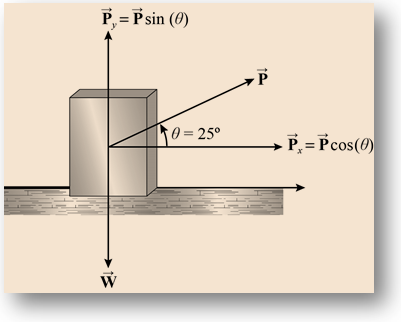Concept:-

The figure below shows the forces acting on the block with mass m:

Assume that the weight of the Block is given by W such that W = mg, the horizontal component of force  is Px = Pcosθ whereas the vertical component of force is  Py = Psinθ (θ is the angle at which the force  acts).

(a) When the block does not lift off from the ground there is no acceleration in the vertical direction. However, the acceleration does exist on the horizontal direction and is given as:

ax = Px/m

Substitute Px = Pcosθ,

ax = Pcosθ/m

Substitute the values of P, m and θ to get the desired result.

(b) When the force  is increased, the magnitude of vertical component of force Py = Psinθ  will also increase. The moment at which the block is about to lift, the magnitude of the vertical component of force will be equal to the weight W, that is,

Py = W

Substitute Py = Psinθ,

Psinθ = W

P = W/sinθ

Substitute the values of W and θ to get the desired result.

(c) Just before the block is lifted there in no acceleration in the vertical direction however the acceleration exists in the horizontal direction, and is given as:

ax = Px/m

Substitute Px = Pcosθ,

ax = Pcosθ/m

Substitute the values of P, m and θ to get the desired result.

Solution:-

(a) Substitute 12 N for P, 5.1 kg  for m and 25º for θ in equation ax = Pcosθ/m,

ax = Pcosθ/m

= 12 N cos (25º) /5.1 kg

= (10.87 N) [(1 kg.m/s2) / 1 N] / 5.1 kg

= 2.2 m/s2

Therefore, the horizontal acceleration of the block is 2.2 m/s2.

(b) The weight of the block is:

W = mg

Substitute 5.1 kg for m and 9.8 m/s2 for g,

W = (5.1 kg) (9.8 m/s2)

= (49.9 kg.m/s2) (1 N/1 kg.m/s2) = 49.9 N

Substitute 49.9 N for W and 25º for θ in equation P = W/sinθ,

P = 49.9 N/sin ( 25º) = 118.26 N

Round off to three significant figures,

P = 119 N

Therefore the magnitude of net force on the block when it is about to lift is 119 N.

(c) Substitute 119 N for P, 5.1 kg for m and 25º for θ in equation ax = Pcosθ/m,

ax= 119 N (cos25º) / 5 kg

= (107.8 N) [(1 kg.m/s2)/1 N] / 5.1 kg = 21.1 m/s2

Round off to two significant figures,

ax = 21 m/s2

Therefore, the acceleration of the block when it is about to lift is 21 m/s2.

_____________________________________________________________________________________________________

Example 2:-

A worker drags a crate across a factory floor by pulling on a rope tied to the crate. The rope, which is inclined at 38.0º above the horizontal, exerts a force of 450 N on the crate. The floor exerts a horizontal resistive force of 125 N, as shown in the below figure. Calculate the acceleration of the crate (a) if its mass is 96.0 kg, and (b) if its weight is 96.0 N.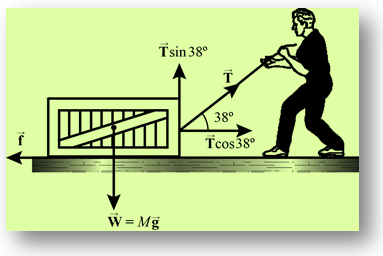Concept:-

The figure below shows the forces acting on the crate of mass M, when it is pulled by a worker. The force experienced by the crate is , and can be resolved into horizontal and vertical components as  and  respectively.

Also assume that the resistive force from the ground is represented by (refer figure above), and the acceleration of the crate in the horizontal direction is given by  and the acceleration in the vertical direction is .

(a) Using Newton’s second law of motion for the forces acting in the horizontal direction, one can write

F cos (38.0º) – f = Max

ax = [F cos (38.0º) – f]/ M                        …… (1)

Therefore, the acceleration of the crate in the horizontal direction is ax = [F cos (38.0º) – f] / M.

Similarly, apply Newton’s second law in the vertical direction,

F sin (38.0º) – Mg = May

ay = F sin (38.0º) – Mg / M

It can be seen from the equation above that if the term F sin (38.0º) is smaller than the weight Mg, the crate will rest on the ground.

(b) If the weight of the crate is W, then its mass is W/g, and the acceleration given by equation (1) can be written as:

a = [F cos (38.0º) – f]/ (W/g)

Therefore, the acceleration of the crate of weight W can be calculated using relation a = [F cos (38.0º) – f]/ (W/g).

Similarly, apply Newton’s second law in the vertical direction,

F sin (38.0º) – W = (W/g) ay

ay = [F sin (38.0º) – W] / [W/g]

Therefore the acceleration of the crate in the vertical direction is ay = [F sin (38.0º) – W] / [W/g].

Solution:-

(a) Substitute 450 N for F, 125 N for f and 96.0 kg for M in equation a = [F cos (38.0º) – f]/ M,

a = [F cos (38.0º) – f]/ M

= [(450 N) cos  (38.0º) – 125 N]/[96.0 kg]

= (229.6 N) [(1 kg.m/s2)/1 N] / 96.0 kg

= 2.40 m/s2

Therefore, the horizontal acceleration of the crate of mass 96.0 kg is 2.40 m/s2.

Substitute 450 N for F in expression F sin (38.0º),

F sin (38.0º) = (450 N) sin (38.0º) = 277 N

Substitute 9.8 m/s2 for g and 96.0 kg for M in expression Mg,

Mg = (96.0 kg) (9.8 m/s2)

= (940.8 kg.m/s2) [1 N/(1 kg.m/s2)] = 940.8 N

The weight of the crate is larger than the upward force F sin (38.0º), therefore the crate will rest on the ground.

(b) Substitute 450 N for F, 125 N for f, 9.8 m/s2 for g and 96.0 N for W in equation a = [F cos (38.0º) – f]/ (W/g),

a = [(450 N) cos (38.0º) – 125 N]/(96.0 N/9.8 m/s2)

= (229.6 N) [(1 kg.m/s2)/1 N] / 9.79 kg = 23.4 m/s2

Therefore, the horizontal acceleration of the crate of weight 96.0 N is 23.4 m/s2.

Substitute 450 N for F, 125 N for f, 9.8 m/s2 for g and 96.0 N for W in equation ay = [F sin (38.0º) – W] / [W/g],

ay = [F sin (38.0º) – W] / [W/g]

= (450 N) sin (38.0º) – (96.0 N)/(96.0 N/9.8 m/s2)

= (181 N) [(1 kg.m/s2)/1 N] / 9.79 kg

= 18.4 m/s2

Therefore, the crate has an upward acceleration of 18.4 m/s2.

____________________________________________________________________________________________________

Example 3:-

A 110-kg crate is pushed at constant speed up the frictionless 34º ramp as shown in the below figure. What horizontal force F is required? (Hint: Resolve forces into components parallel to the ramp.)Concept:-

The figure below shows the component of forces  on the block, moving with a constant speed on a frictionless 34º ramp.

Assume that the x axis is along the length of the ramp, and y axis perpendicular to the ramp such that the component of force along the x axis is Fx = F cos (34º) whereas the component of force along the y axis is Fy = – Fsin (34º).

The block moves up the ramp at constant speed, and therefore has no acceleration in the x direction.

The Newton’s third law of motion for the forces along the x axis gives,

Mg sin (34º) – F cos (34º) = 0

Here M is the mass of the block and g is the acceleration due to gravity.

Mg sin (34º) = F cos (34º)

F = Mg sin (34º) / cos (34º)

= Mg tan (34º)

Therefore the force required to move the block with constant speed on a frictionless 34º ramp is given by relation  F = Mg tan (34º).

Solution:-

Substitute 110 kg for M and 9.8 m/s2 for g in equation F = Mg tan (34º),

F = Mg tan (34º)

= (110 kg) (9.8 m/s2) tan (34º)

= (727 kg.m/s2) [1 N/(1 kg.m/s2)]

= 727 N

Therefore, the magnitude of force required to move the block with constant speed on a frictionless 34º ramp is 727 N.

_________________________________________________________________________________________________

Example 4:-

A jet fighter takes off an angle of 27.0º with the horizontal, accelerating at 2.62 m/s2. The weight of the plane is 79,300 N. Find (a) the thrust T of the engine on the plane and (b) the lift force L exerted by the air perpendicular to the wings; see below figure. Ignore air resistance.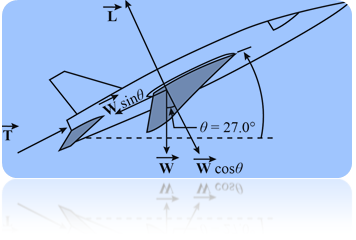Concept:-

The figure below shows the forces acting on the jet fighter:

Assume that the x direction is in the direction of motion of plane during its take off and the y direction perpendicular to it such that the weight W can be resolved into components along the x and y direction as Wx = W sinθ and Wy = W cosθ (θ is the angle between the jet and the ground).

(a) If ax is the acceleration of the jet in x direction, then the net force acting on the jet is F = (W/g) ax (g is the free fall acceleration).

Apply Newton’s second Law of motion along the x direction,

T – Wx = F

Substitute Wx = W sinθ and F = (W/g) ax,

T – Wsinθ = (W/g) ax

T = (W/g) ax + Wsinθ

Substitute the value of ax, W and θ to calculate the desired result.

(b) The jet does not have any acceleration in the y direction, therefore the sum of the forces acting on it in that direction must be equal to zero, that is

L – Wy = 0

L = Wy

Substitute Wy = W cosθ,

L = W cosθ

Solution:-

(a)Substitute 79,300 N for W, 2.62 m/s2 for ax, 9.8 m/s2 for g and 27.0º for θ in equation T = (W/g) ax + Wsinθ,

T = [(79,300 N)/(9.8 m/s2)] (2.62 m/s2) + (79,300 N) sin (27.0º)

= 21,200.6 N + 36001.4 N

= 57,202 N

Round off to three significant figures,

T = 57,000 N

Therefore, the magnitude of thrust T is 57,000 N.

(b) Substitute 79,300 N for W and 27.0º for θ in equation L = W cosθ,

L = W cosθ

= (79,300 N) cos (27.0º)

= 70656.8 N

Round off to three significant figures,

L = 70,600 N

Therefore the magnitude of the force L is 70,600 N.

___________________________________________________________________________________________________

Example 5:-

A certain airplane has a speed of 180 mi/h and is diving at an angle of 27º below the horizontal when a radar decoy is released. The horizontal distance between the release point and the point where the decoy strikes the ground is 2300 ft. (a) How long was the decoy in the air? (b) How high was the plane when the decoy was released? See below figure.Concept:-

The figure below shows the plane, decoy and the initial velocity v0 of the decoy when it was released.

It can be seen from the figure that the horizontal component of velocity of the decoy when it was released is v0 cos (-θ) while the vertical component of the decoy is v0 sin (-θ). It is important to note that the negative sign in θ is due to the fact that the angle is measured in clockwise direction with respect to horizontal.

(a) The time of flight of decoy can be calculated using relation,

t = x/v0cosθ

Here x is the range of the decoy, v0 is the initial velocity and θ is the angle between the decoy with respect to horizontal (refer figure above).

(b) The vertical displacement of the decoy during its time of flight can be calculated using relation,

y = (v0 sinθ) t – ½ gt2

Here g is the acceleration due to gravity and t is the time of flight calculated in part (a).

Solution:-

(a) Substitute -27º for θ, 180 mi/h for v0 and 2300 ft for x in equation t = x/v0cosθ,

t = [2300 ft] / [(180 mi/h) (5280 ft/ 1 mi) (1 h/3600 s) cos (27º)]

= (2300 ft)/(235.2 ft/s)

= 9.77 s

Therefore, it takes 9.77 s for decoy to reach the target.

(b) Substitute 9.77 s for t, 32 ft/s2 for g, -27º for θ and 180 mi/h for v0 in equation y = (v0 sinθ) t – ½ gt2 ,

y = ((180 mi/h) sin (27º)) (9.77 s) – ½ (32 ft/s2) (9.77 s)2

= [((180 mi/h) (5280 ft/1 mi) (1 h/3600 s) sin (-27º)) (9.77 s)] – ½ (32 ft/s)2 (9.77 s)2

= -1170.9 ft – 15272 ft

= -2698 ft

The negative sign accounts for the fact that the decoy moves closer to ground.

Therefore, the vertical displacement of decoy is 2698 ft.

___________________________________________________________________________________________________

Example 6:-

In earlier days, horses pulled barges down canals in the manner shown in below figure. Suppose that the horse pulls a rope that exerts a horizontal force of 7900 N at an angle of 18º to the direction of motion of the barge, which is headed straight along the canal. The mass of the barge, which is headed straight along the canal. The mass of the barge is 9500 kg and its acceleration is 0.12 m/s2. Calculate the horizontal force exerted by the water on the barge.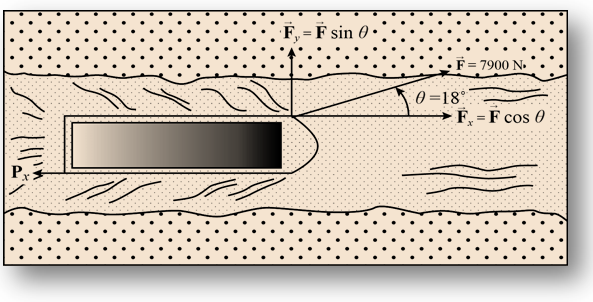Concept:-

The force say  acting on the barges of mass m is show in the figure below:

The horizontal component of force on the barge is Fx = F cosθ whereas the vertical component of force is Fy = F sinθ (θ is the angle made by force F relative to horizontal axis).

Assume that the horizontal force exerted by the water on the barge is Px, then from Newton’s second law one can write,

Fx – Px = max

Px = Fx - max

Here, ax is the acceleration of the barges in the horizontal direction.

Substitute

Fx = F cosθ,

Px = F cosθ - max

Substitute the value of F, m and a to get the desired result.

Solution:-

Substitute 0.12 m/s2 for a, 9500 kg for m, 7900 N for F and 18º for θ in equation Px = F cosθ - max,

Px = F cosθ - max

= (7900 N) cos(18º) – (9500 kg) (0.12 m/s2)

= 7513.3 N – (1140 kg.m/s2) [1 N/(1 kg.m/s2)]

= 6373. 3 N

Round off to four significant figures,

Px = 6373 N

Therefore the magnitude of horizontal force exerted by the water is 6373 N and it acts in a direction opposite to the horizontal motion of barges.

__________________________________________________________________________________________

Example 7:-

A dart is thrown horizontally toward the bull’s eye, point P on the dart board, with an initial speed of 10 m/s. It hits at point Q on the rim, vertically below P, 0.19 s later; as shown in below figure. (a) What is the distance PQ? (b) How far away from the dart board did the player stand?Concept:-

(a) The distance PQ is the vertical distance travelled by the dart in time t under the action of gravitational force, and can be given as:

y = - ½ gt2

The negative sign is due to the convention that the vectors pointing downwards are taken as negative.

Here g is the acceleration due to gravity and t is the elapsed time.

(b) The horizontal distance between the dart board and the player can be calculated using relation,

x = v0xt

Here v0x is the initial speed of the dart and t is the elapsed time.

Solution:-

(a)Substitute 9.81 m/s2 for g and 0.19 s for t in equation y = - ½ gt2,

y = - ½ (9.81 m/s2) (0.19 s)2

= - 0.17 m

The negative sign indicates the fact that the displacement is in the negative y direction.

Therefore the distance PQ is -0.17 m.

(b) Substitute 0.19 s for t and 10 m/s for v0x in equation x = v0xt,

x = (10 m/s) (0.19 s)

= 1.9 m

Therefore, the horizontal separation between the dart board and the player is 1.9 m.

_________________________________________________________________________________________

Example 8:-

In a baseball game, a batter hits the ball at a height of 4.60 ft above the ground so that its angle of projection is 52.0º to the horizontal. The ball lands in the grandstand, 39.0 ft up from the bottom; see in below figure. The grandstand seats slope upward at 28.0º with the bottom seats 358 ft from home plate. Calculate the speed with which the ball left the bat. (Ignore air resistance.)Concept:-

The figure below shows the trajectory of the ball:

It can be seen from the figure above that the total horizontal displacement say x of the ball is x = 358 ft + 39 ft cos (28º) whereas the vertical displacement say y of the ball is y =39 ft sin (28º) – 4.6 ft .

Assume that the ball left the ball with initial velocity v0 at an angle θ such that the horizontal component of initial velocity is v0x = v0 cosθ whereas the vertical component of initial velocity is  v0y = v0 sinθ.

The ball does not accelerate in the horizontal direction, therefore the horizontal distance travelled by the ball is:

x = v0xt

Substitute x = 358 ft + 39 ft cos (28º)  and vox = v0cosθ,

358 ft + 39 ft cos (28º) = (v0cosθ) t

t = [358 ft + 39 ft cos (28º)] / (v0cosθ)

Similarly, the vertical displacement of the ball can be written as:

y = v0yt – ½ gt2

Substitute v0y = v0 sinθ and y =39 ft sin (28º) – 4.6 ft,

[39 ft sin (28º) – 4.6 ft] = (v0 sinθ)t – ½ gt2

Substitute t = [358 ft + 39 ft cos (28º)] / (v0cosθ) ,

[39 ft sin (28º) – 4.6 ft] = (v0 sinθ)[[358 ft + 39 ft cos (28º)] / (v0cosθ)]– ½ g{[358 ft + 39 ft cos (28º)] / (v0cosθ)}2

13.7 ft = (392.4 ft) tanθ – ½ g (392.4 ft/v0cosθ)2

Solve the equation for v0 to get the desired result.

Solution:-

Substitute 52º for θ and 32.2 ft/s2 for g in equation 13.7 ft = (392.4 ft) tanθ – ½ g (392.4 ft/v0cosθ)2,

13.7 ft = (392.4 ft) tan (52º) – ½ g (392.4 ft/v0cos(52º))2,

½ (32.2 ft/s2) [392.4 ft/v0 cos (52º)]2 = 488.5 ft

Round of to three significant figures,

v0 = 115 ft/s

Therefore, the speed with which the ball leaves the bat is 115 ft/s.

_____________________________________________________________________________________________

Example 9:-

You throw a ball with a speed of 25.3 m/s at an angle of 42.0º above the horizontal directly toward a a wall as shown in below figure. The wall is 21.8 m from the release point of the ball. (a) How long is the ball in the air before it hits the wall? (b) How far above the release point does the ball hit the wall? (c) What are the horizontal and vertical components of its velocity as it hits the wall? (d) Has it passed the heighest point on its trajectory when it hits?

Concept:-

The figure below shows the velocity of the ball and its components along the horizontal and vertical directions respectively: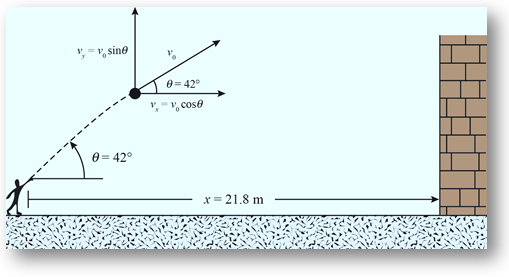The horizontal component of initial velocity of ball is:

vx = v0 cosθ

Here, v0 is the magnitude of initial velocity of the ball, and θ is the angle at which the ball is projected relative to horizontal.

The vertical component of initial velocity of the ball is:

vy = v0 sinθ

Here, v0 is the magnitude of initial velocity of the ball, and θ is the angle at which the ball is projected relative to horizontal.

(a) The time of flight of the ball is given as:

t = x/vx

Here, x is the range of the projectile.

Substitute vx = v0 cosθ,

t = x/v0 cosθ

Substitute the value of x, v0 and θ to get the desired result.

(b) The vertical displacement of the ball corresponding to time t is:

y = vyt – ½ gt2

Substitute vy = v0sinθ,

y = (v0sinθ) t – ½ gt2

Substitute the calculated value of t, and the given values of v0, θ to obtain the desired result.

(c) The horizontal component of velocity remains the same as the ball hits the wall because the ball has no acceleration in the horizontal direction. However, the ball acceleration in the vertical direction and therefore the magnitude of its final vertical velocity changes to,

vy’ = vy – gt

Substitute vy = v0 sin θ,

vy’ = v0 sin θ – gt

Substitute the calculated value of t, and the given values of v0, θ and g to obtain the desired result.

(d) If the magnitude of vy’ has a positive sign, the ball is still heading up and has not reached its maximum height yet. However, if the sign is negative, the velocity vector of the ball has reversed its direction and the ball has started falling downwards depicting that the maximum height is being crossed.

Solution:-

(a) Substitute 25.3 m/s for v0, 42.0º for θ and 21.8 m for x in equation t = x/v0 cosθ,

t = [21.8 m]/[(25.3 m/s) cos (42.0º)] = 1.15 s

Therefore, the time of flight of the ball is 1.15 s.

(b) Substitute 25.3 m/s for v0, 42.0º for θ, 9.8 m/s2 for g, and 1.15 s for t in equation y = (v0sinθ) t – ½ gt2,

y = (v0sinθ) t – ½ gt2

= ((25.3 m/s) sin (42.0º)) (1.15 s) – ½ (9.8 m/s)2 (1.15 s)2 = 12.9 m

Round off to two significant figures,

y = 13 m

Therefore, the vertical distance travelled by the ball in 1.15 s is 13 m.

(c) Substitute 25.3 m/s for v0, 42.0º for θ, 9.8 m/s2 for g, and 1.15 s for t  in equation vy’ = v0 sin θ – gt,

vy’ = v0 sin θ – gt

= (25.3 m/s) sin (42.0º) – (9.8 m/s2) (1.15 s)

= 5.65 m/s

Therefore, the vertical component of final velocity of the ball before hitting the wall is 5.65 m/s.

The horizontal component of velocity remains unchanged during its flight,

vx’ = vx = v0 cosθ

Substitute 25.3 m/s for v0 and 42.0º for θ,

vx’ = (25.3 m/s) cos (42.0º) = 18.8 m/s

Therefore, the horizontal component of final velocity of ball, before hitting the wall is 18.8 m/s.

(d) From part (c) it is clear that vertical component of final velocity of ball is 5.65 m/s, and is positive. Therefore the ball is yet to reach its maximum height but collided with the wall before doing the same.

_____________________________________________________________________________________________

Example 10:-

(a) In Galileo’s Two New Sciences, the author states that “for elevations [ angles of projection] which exceed or fall short of 45º by equal amounts, the ranges are equal.” Prove this statement. See below figure. (b) For an initial speed of 30.0 m/s and a range of 20.0 m, find the two possible elevation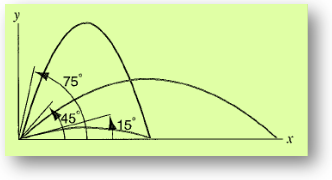Concept:-

Assume the angle by which one of the projectiles falls shorter of 45º is θ whereas the other one exceeds 45º by the same account.

The range of the projectile with angle of projection 45º – θ  is given as:

R1 = v02 sin ( 45º – θ) / g

Here, v0 is the initial speed of the projectile, and g is the acceleration due to gravity.

Similarly, the range of the projectile with angle of projection 45º + θ is:

R2 = v02 sin 2 ( 45º + θ) / g

Here, v0 is the initial speed of the projectile, and g is the acceleration due to gravity.

The term v02/ g remains the same in R1 and R2, therefore if the values sin 2 (45º - θ) is equal to sin 2 (45º - θ), then the range R1 and R2 is equal.

(b) Assume the angle of projection of one of the projectile is θ, then its value can be calculated from expression for R as

R = v02 sin 2θ / g

θ = ½ sin-1 (Rg/v02)

The angle of projection for the other projectile is 90º - θ.

Solution:-

(a) The quantities  sin 2 (45º - θ) and  sin 2 (45º + θ) can be written as sin 2 (45º± θ).

Also,

sin 2 (45º± θ) = sin (90º± 2θ)

= cos (± 2θ)

= cos (2θ)

It is important to note that cos (± 2θ) = cos (-2θ) = cos (θ), and therefore the terms sin 2 (45º - θ)  and sin 2 (45º + θ) are equal. This proves the range R1 is equal to range R2.

(b) Substitute 30 m/s for v0, 20.0 m for R and 9.8 m/s2 for g in equation θ = ½ sin-1 (Rg/v02),

θ = ½ sin-1[(20.0 m) (9.8 m/s2) / (30 m/s)2] = 6.28º

Round off to two significant figures,θ = 6.3º

The other possible elevation angle of projectile is:

? = 90º – θ

Substitute θ = 6.3º,

? = 90º – 6.3º

= 83.7º

Therefore, the first possible elevation angle of projectile is 6.3º, whereas the other possible elevation angle of projectile is 83.7º.

Related Resources:-

You might like to refer Newton’s Third Law of Motion.

For getting an idea of the type of questions asked, refer the  Previous Year Question Papers.

To read more, Buy study materials of Kinematics comprising study notes, revision notes, video lectures, previous year solved questions etc. Also browse for more study materials on Physics here.### Course Features

• 101 Video Lectures
• Revision Notes
• Previous Year Papers
• Mind Map
• Study Planner
• NCERT Solutions
• Discussion Forum
• Test paper with Video Solution